## Properties of Fluid in Fluid Mechanics:

The following properties of fluids are of general importance to the study of fluid mechanics.

• Mass density (ρ)
• Specific Volume (v)
• Specific weight (w)
• Specific gravity (S)

#### 1. Mass density (ρ):

Mass density or Density is defined as the mass per unit volume i.e., mass of the fluid contained in 1m3 volume. It is denoted by a Greek symbol ρ (rho).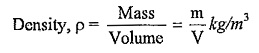Its unit is kg/m3. Density decreases with increase in temperature and it increases with increase in pressure. The characteristic equation is used to estimate the density from the measurement of pressure, temperature and volume.

The characteristic equation is pV = mRT. Density of water is 1000kg/m3 at a standard condition.

#### 2. Specific Volume (v):

It is defined as volume of fluid occupied by unit mass.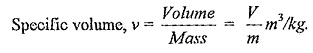The unit used is m3/kg. It is the reciprocal of density.

#### 3. Specific weight (w):

It is defined as the weight possesses per unit volume. It is denoted by w. It varies from place to place because of acceleration due to gravity varies from place to place.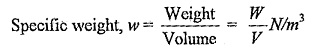It decreases with increase in temperature and increases with increase pressure. Mathematically, it can also be denoted by w = ρg.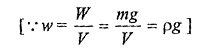is also known as “weight density”.

#### 4. Specific gravity (S):

It is the ratio of specific weight of a any fluid to the specific weight of standard fluid. It can also be defined as the ratio of mass density of the given fluid to the mass density of the standard fluid.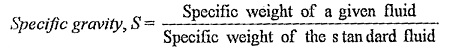or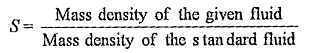For liquids the standard Properties of Fluid choosen for comparison is pure water at specific gravity of water at 4°C is taken as 1.0. Gases, hydrogen or air is choosen.

Specific weight of given liquid = Specific gravity of liquid x Specific weight of water

= S x 9.81N/m3.

Density of the given liquid = Specific gravity of liquid x Density of water

= S x 1000kg/m3

Scroll to Top